GATE  >  MA Mathematics - 2013 GATE Paper (Practice Test)

# MA Mathematics - 2013 GATE Paper (Practice Test)

Test Description

## 65 Questions MCQ Test GATE Past Year Papers for Practice (All Branches) | MA Mathematics - 2013 GATE Paper (Practice Test)

MA Mathematics - 2013 GATE Paper (Practice Test) for GATE 2023 is part of GATE Past Year Papers for Practice (All Branches) preparation. The MA Mathematics - 2013 GATE Paper (Practice Test) questions and answers have been prepared according to the GATE exam syllabus.The MA Mathematics - 2013 GATE Paper (Practice Test) MCQs are made for GATE 2023 Exam. Find important definitions, questions, notes, meanings, examples, exercises, MCQs and online tests for MA Mathematics - 2013 GATE Paper (Practice Test) below.
Solutions of MA Mathematics - 2013 GATE Paper (Practice Test) questions in English are available as part of our GATE Past Year Papers for Practice (All Branches) for GATE & MA Mathematics - 2013 GATE Paper (Practice Test) solutions in Hindi for GATE Past Year Papers for Practice (All Branches) course. Download more important topics, notes, lectures and mock test series for GATE Exam by signing up for free. Attempt MA Mathematics - 2013 GATE Paper (Practice Test) | 65 questions in 180 minutes | Mock test for GATE preparation | Free important questions MCQ to study GATE Past Year Papers for Practice (All Branches) for GATE Exam | Download free PDF with solutions
 1 Crore+ students have signed up on EduRev. Have you?
MA Mathematics - 2013 GATE Paper (Practice Test) - Question 1

### Q. 1 – Q. 5 carry one mark each. Q. A number is as much greater than 75 as it is smaller than 117. The number is:

MA Mathematics - 2013 GATE Paper (Practice Test) - Question 2

###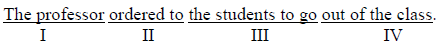Which of the above underlined parts of the sentence is grammatically incorrect?

MA Mathematics - 2013 GATE Paper (Practice Test) - Question 3

### Which of the following options is the closest in meaning to the word given below: HistoricPrimeval

MA Mathematics - 2013 GATE Paper (Practice Test) - Question 4

Friendship, no matter how _________it is, has its limitations

MA Mathematics - 2013 GATE Paper (Practice Test) - Question 5

Select the pair that best expresses a relationship similar to that expressed in the pair: Medicine: Health

MA Mathematics - 2013 GATE Paper (Practice Test) - Question 6

Q. 6 to Q. 10 carry two marks each.

Q. X and Y are two positive real numbers such that 2X + Y ≤ 6 and X + 2Y ≤ 8. For which of the following values of (X, Y) the function /(X, Y) = 3X + 6Y will give maximum value?

MA Mathematics - 2013 GATE Paper (Practice Test) - Question 7

If |4X−7|=5 then the values of 2 |X|− |−X| is:

MA Mathematics - 2013 GATE Paper (Practice Test) - Question 8

Following table provides figures (in rupees) on annual expenditure of a firm for two years - 2010 and 2011.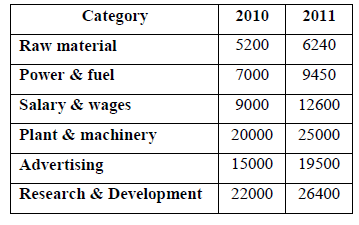In 2011, which of the following two categories have registered increase by same percentage?

MA Mathematics - 2013 GATE Paper (Practice Test) - Question 9

A firm is selling its product at Rs. 60 per unit. The total cost of production is Rs. 100 and firm is earning total profit of Rs. 500. Later, the total cost increased by 30%. By what percentage the price should be increased to maintained the same profit level.

MA Mathematics - 2013 GATE Paper (Practice Test) - Question 10

Abhishek is elder to Savar. Savar is younger to Anshul. Which of the given conclusions is logically valid and is inferred from the above statements?

MA Mathematics - 2013 GATE Paper (Practice Test) - Question 11

Q. 11 – Q. 35 carry one mark each.

Q. The possible set of eigen values of a 4 × 4 skew-symmetric orthogonal real matrix is

MA Mathematics - 2013 GATE Paper (Practice Test) - Question 12

The coefficient of (z−π)2 in the Taylor series expansion of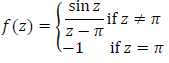around π is

MA Mathematics - 2013 GATE Paper (Practice Test) - Question 13

Consider R2 with the usual topology. Which of the following statements are TRUE for all A,B ⊆ R2?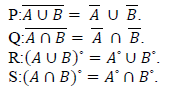*Answer can only contain numeric values
MA Mathematics - 2013 GATE Paper (Practice Test) - Question 14

Let f:R →R be a continuous function with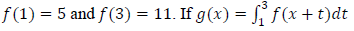then  g′ (0) is equal to ______

(Important : you should answer only the numeric value)

*Answer can only contain numeric values
MA Mathematics - 2013 GATE Paper (Practice Test) - Question 15

Let P be a 2×2 complex matrix such that trace(P)=1 anddet(P)=−6. Then, trace(P4−P3) is ______

(Important : you should answer only the numeric value)

MA Mathematics - 2013 GATE Paper (Practice Test) - Question 16

Suppose that R is a unique factorization domain and that a,b ∈ R are distinct irreducible elements. Which of the following statements is TRUE?

MA Mathematics - 2013 GATE Paper (Practice Test) - Question 17

Let X be a compact Hausdorff topological space and let Y be a topological space. Let f:X→Y be a bijective continuous mapping. Which of the following is TRUE?

MA Mathematics - 2013 GATE Paper (Practice Test) - Question 18

Consider the linear programming problem: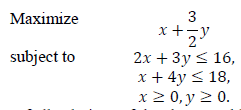If S denotes the set of all solutions of the above problem, then

MA Mathematics - 2013 GATE Paper (Practice Test) - Question 19

Which of the following groups has a proper subgroup that is NOT cyclic?

*Answer can only contain numeric values
MA Mathematics - 2013 GATE Paper (Practice Test) - Question 20

The value of the integral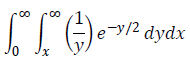is _________________

(Important : you should answer only the numeric value)

MA Mathematics - 2013 GATE Paper (Practice Test) - Question 21

Suppose the random variable u has uniform distribution on [0,1] and X=−2logu. The density of X is

MA Mathematics - 2013 GATE Paper (Practice Test) - Question 22

Let f be an entire function on C such that |f(z)|≤100log|z|for each z with|z|≥2. If f(i)=2i, then f(1)

*Answer can only contain numeric values
MA Mathematics - 2013 GATE Paper (Practice Test) - Question 23

The number of group homomorphisms from Z3 to Z9 is ______

(Important : you should answer only the numeric value)

*Answer can only contain numeric values
MA Mathematics - 2013 GATE Paper (Practice Test) - Question 24

Let u(x,t) be the solution to the wave equation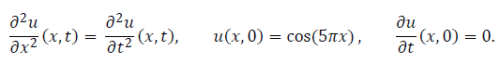Then, the value of u(1,1) is ______

(Important : you should answer only the numeric value)

MA Mathematics - 2013 GATE Paper (Practice Test) - Question 25

Let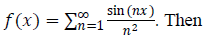MA Mathematics - 2013 GATE Paper (Practice Test) - Question 26

Suppose X is a random variable with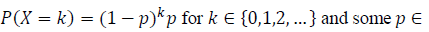(0,1). For the hypothesis testing problem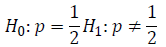consider the test “Reject H0 if X ≤ A or if X ≥ B”, where A < B are given positive integers. The type-I error of this test is

*Answer can only contain numeric values
MA Mathematics - 2013 GATE Paper (Practice Test) - Question 27

Let G be a group of order 231. The number of elements of order 11 in G is ______

(Important : you should answer only the numeric value)

MA Mathematics - 2013 GATE Paper (Practice Test) - Question 28

Let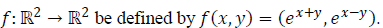The area of the image of the region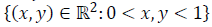under the mapping f is

MA Mathematics - 2013 GATE Paper (Practice Test) - Question 29

Which of the following is a field?

MA Mathematics - 2013 GATE Paper (Practice Test) - Question 30

Let x0=0. Define xn+1=cosxn for every n≥0. Then

MA Mathematics - 2013 GATE Paper (Practice Test) - Question 31

Let C be the contour |Z|=2 oriented in the anti-clockwise direction. The value of the integral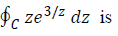MA Mathematics - 2013 GATE Paper (Practice Test) - Question 32

For each λ>0, let Xλ be a random variable with exponential density λe−λx on(0,∞). Then, Var(logXλ)

MA Mathematics - 2013 GATE Paper (Practice Test) - Question 33

Let {an} be the sequence of consecutive positive solutions of the equation tanx=x and let {bn} be the sequence of consecutive positive solutions of the equationtan√x=x. Then

MA Mathematics - 2013 GATE Paper (Practice Test) - Question 34

Let f be an analytic function on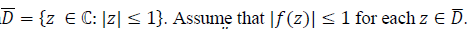Then, which of the following is NOT a possible value of (ef)′′ (0)?

*Answer can only contain numeric values
MA Mathematics - 2013 GATE Paper (Practice Test) - Question 35

The number of non-isomorphic abelian groups of order 24 is ______

(Important : you should answer only the numeric value)

*Answer can only contain numeric values
MA Mathematics - 2013 GATE Paper (Practice Test) - Question 36

Q. 36 to Q. 65 carry two marks each.

Q.

Let V be the real vector space of all polynomials in one variable with real coefficients and having degree at most 20. Define the subspaces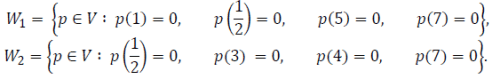Then the dimension of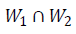is ________________

(Important : you should answer only the numeric value)

MA Mathematics - 2013 GATE Paper (Practice Test) - Question 37

Let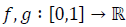be defined by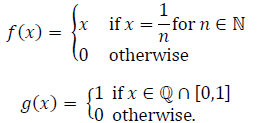Then

*Answer can only contain numeric values
MA Mathematics - 2013 GATE Paper (Practice Test) - Question 38

Consider the following linear programming problem: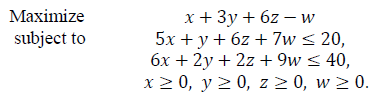Then the optimal value is ______

(Important : you should answer only the numeric value)

MA Mathematics - 2013 GATE Paper (Practice Test) - Question 39

Suppose X is a real-valued random variable. Which of the following values CANNOTbe attained by E[X] and E[X2], respectively?

MA Mathematics - 2013 GATE Paper (Practice Test) - Question 40

Which of the following subsets of R2 is NOT compact?

*Answer can only contain numeric values
MA Mathematics - 2013 GATE Paper (Practice Test) - Question 41

Let M be the real vector space of 2×3 matrices with real entries. Let T:M→M be defined by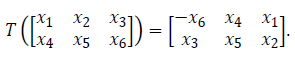The determinant of T is ______

(Important : you should answer only the numeric value)

MA Mathematics - 2013 GATE Paper (Practice Test) - Question 42

Let H be a Hilbert space and let {en:n ≥ 1} be an orthonormal basis of H. Suppose T:H→ H is a bounded linear operator. Which of the following CANNOT be true?

MA Mathematics - 2013 GATE Paper (Practice Test) - Question 43

The value of the limit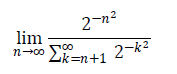is

MA Mathematics - 2013 GATE Paper (Practice Test) - Question 44

Let f: C{3i}→C be defined by f(z)=z−i/(iz +3) . Which of the following statements about f is FALSE?

MA Mathematics - 2013 GATE Paper (Practice Test) - Question 45

The matrix A=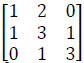can be decomposed uniquely into the product A=LU , where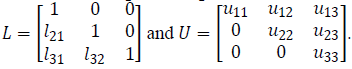The solution of the system LX = [1 2 2]t is

MA Mathematics - 2013 GATE Paper (Practice Test) - Question 46

Let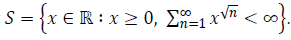Then the supremum of S is

MA Mathematics - 2013 GATE Paper (Practice Test) - Question 47

The image of the region {z ∈C: Re(z)>Im(z)>0} under the mapping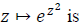MA Mathematics - 2013 GATE Paper (Practice Test) - Question 48

Which of the following groups contains a unique normal subgroup of order four?

(Note: correct answer will be updated soon, Temporary marked A)

MA Mathematics - 2013 GATE Paper (Practice Test) - Question 49

Let B be a real symmetric positive-definite n×n matrix. Consider the inner product on Rn defined by =YtBX . Let A be an n × n real matrix and let T:Rn→Rn be the linear operator defined by T(X)=AX for all X ∈ Rn. If S is the adjoint of T,then S(X)=CX for all X∈Rn,where C is the matrix

MA Mathematics - 2013 GATE Paper (Practice Test) - Question 50

Let X be an arbitrary random variable that takes values in{0,1,…,10}. The minimum and maximum possible values of the variance of X are

MA Mathematics - 2013 GATE Paper (Practice Test) - Question 51

Let M be the space of all 4x3 matrices with entries in the finite field of three elements. Then the number of matrices of rank three in M is

MA Mathematics - 2013 GATE Paper (Practice Test) - Question 52

Let V be a vector space of dimension m≥2. Let T:V→V be a linear transformation such that Tn+1=0 and Tn≠0 for some n≥1. Then which of the following is necessarily TRUE?

MA Mathematics - 2013 GATE Paper (Practice Test) - Question 53

Let X be a convex region in the plane bounded by straight lines. Let X have 7 vertices. Suppose F(x,y)=ax + by + c has maximum valueM and minimum valueN on X and N < M. Let S = {P: P is a vertex of X and N < f (P) <M}. If S has n elements, then which of the following statements is TRUE?

MA Mathematics - 2013 GATE Paper (Practice Test) - Question 54

Which of the following statements are TRUE?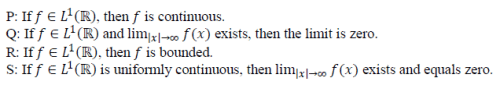MA Mathematics - 2013 GATE Paper (Practice Test) - Question 55

Let u be a real valued harmonic function on C. Let g:R2→R be defined by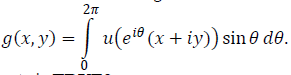Which of the following statements is TRUE?

MA Mathematics - 2013 GATE Paper (Practice Test) - Question 56

Let S={Z∈C: |Z|=1} with the induced topology from C and let f:[0,2]→S be defined as f(t)=e2πit . Then, which of the following is TRUE?

MA Mathematics - 2013 GATE Paper (Practice Test) - Question 57

Assume that all the zeros of the polynomial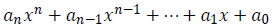have negative real parts. If u(t) is any solution to the ordinary differential equation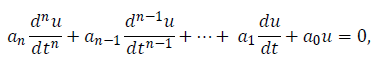then limt→∞ u(t) is equal to

MA Mathematics - 2013 GATE Paper (Practice Test) - Question 58

Common Data for Questions 58 and 59:

Let C00 be the vector space of all complex sequences having finitely many non-zero terms. Equip c00 with the inner product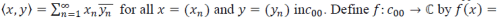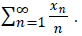Let N be the kernel of f

Q. Which of the following is FALSE?

MA Mathematics - 2013 GATE Paper (Practice Test) - Question 59

Which of the following is FALSE?

MA Mathematics - 2013 GATE Paper (Practice Test) - Question 60

Common Data for Questions 60 and 61:

Let X1,X2,…,Xn be an i.i.d. random sample from exponential distribution with mean μ. In other words, they have density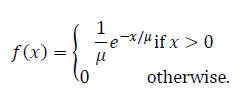Q.

Which of the following is NOT an unbiased estimate of μ?

MA Mathematics - 2013 GATE Paper (Practice Test) - Question 61

Let X1,X2,…,Xn be an i.i.d. random sample from exponential distribution with mean μ. In other words, they have densityQ.

Consider the problem of estimating μ. The m.s.e (mean square error) of the estimate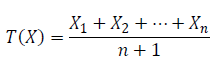is

*Answer can only contain numeric values
MA Mathematics - 2013 GATE Paper (Practice Test) - Question 62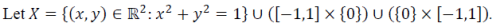Let n0=max { k : k< ∞, there are k distinct points p1,…,p∈ X such that x{p1,…,pk} is connected}

Q.

The value of n0 is ______

(Important : you should answer only the numeric value)

*Answer can only contain numeric values
MA Mathematics - 2013 GATE Paper (Practice Test) - Question 63Let n0=max { k : k< ∞, there are k distinct points p1,…,p∈ X such that x{p1,…,pk} is connected}

Q.

Let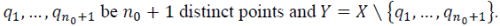Let m be the number of connected components of Y. The maximum possible value of m is ______

(Important : you should answer only the numeric value)

MA Mathematics - 2013 GATE Paper (Practice Test) - Question 64

Let Let W(y1,y2) be the Wrönskian of two linearly independent solutions y1 and y2 of the equation y′′+P(x)y′+Q(x)y=0.

Q.

The product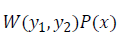equals

MA Mathematics - 2013 GATE Paper (Practice Test) - Question 65

Let Let W(y1,y2) be the Wrönskian of two linearly independent solutions y1 and y2 of the equation y′′+P(x)y′+Q(x)y=0.

Q.

If y1=e2x and y2=e2x, then the value of P(0) is

## GATE Past Year Papers for Practice (All Branches)

407 docs|127 tests
 Use Code STAYHOME200 and get INR 200 additional OFF Use Coupon Code
Information about MA Mathematics - 2013 GATE Paper (Practice Test) Page
In this test you can find the Exam questions for MA Mathematics - 2013 GATE Paper (Practice Test) solved & explained in the simplest way possible. Besides giving Questions and answers for MA Mathematics - 2013 GATE Paper (Practice Test), EduRev gives you an ample number of Online tests for practice

## GATE Past Year Papers for Practice (All Branches)

407 docs|127 tests